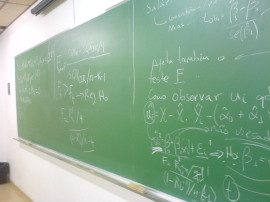﻿

# Seminario 20/13: Joscea Krause (Universität Trier). Regularized Small Area Estimation: A Framework for Robust Estimates in the Presence of Unknown Measurement Errors

### Información

• Ponente: Joscea Krause, Universität Trier.
• Fecha: 01/Jun/2020 - 12:00 horas
• Lugar: SEMINARIOS ONLINE CIO (UMH): http://cio.edu.umh.es/seminariosonline/ (Se grabará)SAE provides stable estimates of area statistics in the presence of small samples. This is achieved by combining observations from multiple areas in suitable regression models.These models exploit the functional relation between the area statistic and contextually related covariate data to make predictions for the quantities of interest. An important assumption of this methodologyis that the covariate data is measured correctly. If this does not hold, areastatistic estimates can be severely biased or highly inefficient. In that case, methodological adjustments are required to allow for reliable results. There are several approachesin the literature that allow for robust estimates from contaminated data bases. Unfortunately, many of them share a common limitation. Robust SAE techniques typically require distribution assumptions on the measurement error. Theseassumptions can be either explicit by requiring a specific distribution, or implicit by demanding that the distribution is known. However, both settings are rarely verifiable in practice. We propose a new approach torobust SAE that does not require distribution assumptions on the measurement error. Using insights into robust optimization theory, we proof that regularized model parameter estimation is equivalent to the robust minimization of loss functions under arbitrary model matrix perturbations. This equivalence holds for many well-established regularized regression methods, such as theLASSO, ridge regression, and the elastic net. It allows us to produce reliablearea statistic estimates in the presence of unknown covariate measurement errors. We built upon this result toderive a modified Jackknife algorithm that allows for conservative MSEestimation for predictions obtained on contaminated data bases. In addition to that, we discuss consistency in model parameter estimation of regularized regression in this setting. The effectiveness of the methodology is demonstrated in a Monte Carlo simulation study.